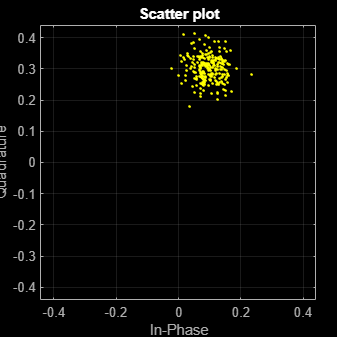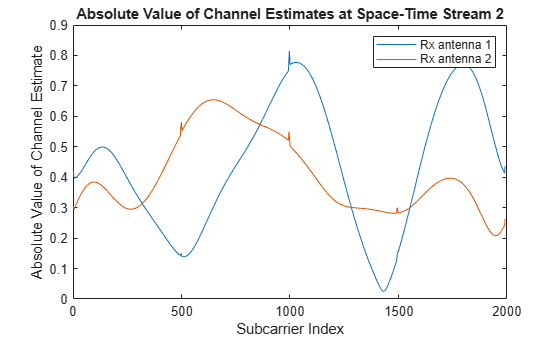# wlanHELTFChannelEstimate

Channel estimation using HE-LTF

## Syntax

``chEst = wlanHELTFChannelEstimate(demodSig,cfg)``
``chEst = wlanHELTFChannelEstimate(demodSig,cfg,ruNumber)``
``[chEst,chEstPilots] = wlanHELTFChannelEstimate(___)``
``[chEst,chEstPilots] = wlanHELTFChannelEstimate(___,FrequencySmoothingSpan=span)``

## Description

example

````chEst = wlanHELTFChannelEstimate(demodSig,cfg)` returns the channel estimate at the high efficiency long training field (HE-LTF) using the demodulated HE-LTF signal, `demodSig`, given the parameters specified in the configuration object `cfg`. For more information about this field, see HE-LTF.```
````chEst = wlanHELTFChannelEstimate(demodSig,cfg,ruNumber)` returns the channel estimate for the resource unit (RU) of interest for a multi-user (MU) configuration. Here, `cfg` must be specified as a `wlanHEMUConfig` object.```

example

````[chEst,chEstPilots] = wlanHELTFChannelEstimate(___)` returns the channel estimate at each pilot subcarrier location for each demodulated HE-LTF OFDM symbol in addition to any input argument combination from the previous syntaxes.```

example

````[chEst,chEstPilots] = wlanHELTFChannelEstimate(___,FrequencySmoothingSpan=span)` specifies frequency smoothing using a name-value pair argument. ```

## Examples

collapse all

Calculate and plot the channel estimate of an HE SU format channel by using the high efficiency long training field.

Create an HE SU format configuration object. Generate a time-domain waveform for an 802.11ax packet.

```cfg = wlanHESUConfig; txSig = wlanWaveformGenerator([1;0;0;1],cfg);```

Multiply the transmitted signal by 0.1 + 0.3i and pass it through an AWGN channel with a signal-to-noise ratio of 25 dB.

`rxSig = awgn((0.1 + 0.3i)*txSig,25);`

Extract the HE-LTF field indices and demodulate the HE-LTF. Perform channel estimation without frequency smoothing.

```idxHELTF = wlanFieldIndices(cfg,"HE-LTF"); demodSig = wlanHEDemodulate(rxSig(idxHELTF(1):idxHELTF(2),:),"HE-LTF",cfg); est = wlanHELTFChannelEstimate(demodSig,cfg);```

Plot the channel estimate.

```scatterplot(est) grid```The channel estimate matches the complex channel multiplier.

Calculate and plot the channel estimate of an HE SU format MIMO channel by using the high efficiency long training field.

Create an HE SU format configuration format object, with a channel bandwidth of 160 MHz and two transmit antennas and two space-time streams. Generate a time-domain waveform for an 802.11ax packet.

```cfg = wlanHESUConfig(NumSpaceTimeStreams=2, NumTransmitAntennas=2); cfg.ChannelBandwidth = "CBW160"; txSig = wlanWaveformGenerator([1;0;0;1],cfg);```

Create a TGax channel system object with a channel bandwidth of 160 MHz and two transmit and receive antennas. Pass the transmitted signal through the TGax MIMO channel.

```tgax = wlanTGaxChannel; tgax.ChannelBandwidth = "CBW160"; tgax.NumReceiveAntennas = 2; tgax.NumTransmitAntennas = 2; rxSig = tgax(txSig);```

Extract the HE-LTF field indices and demodulate the HE-LTF. Using a filter span of 7, perform channel estimation at each pilot subcarrier location with frequency smoothing.

```idxHELTF = wlanFieldIndices(cfg,"HE-LTF"); demodSig = wlanHEDemodulate(rxSig(idxHELTF(1):idxHELTF(2),:),"HE-LTF",cfg); [est,estPilots] = wlanHELTFChannelEstimate(demodSig,cfg,FrequencySmoothingSpan=7);```

Plot the absolute value of the channel estimate for the second of the two space-time streams at both receive antennas.

```plot(abs(est(:,2,1))) hold on plot(abs(est(:,2,2))) xlabel("Subcarrier Index") title("Absolute Value of Channel Estimates at Space-Time Stream 2") ylabel("Absolute Value of Channel Estimate") legend("Rx antenna 1", "Rx antenna 2")```## Input Arguments

collapse all

Demodulated HE-LTF signal, specified as an NST-by-NSYM-by-NR array. NST is the number of occupied subcarriers, NSYM is the number of demodulated HE-LTF OFDM symbols, and NR is the number of receive antennas.

Data Types: `single` | `double`
Complex Number Support: Yes

RU number in an HE MU transmission, specified as a positive integer. The RU number specifies the location of the RU within the channel. For example, consider an 80 MHz transmission with two 242-tone RUs and one 484-tone RU, in order of absolute frequency. For this allocation:

• RU number 1 corresponds to the 242-tone RU in the 20 MHz subchannel at the lowest absolute frequency (size 242, index 1).

• RU number 2 corresponds to the 242-tone RU in the 20 MHz subchannel at the next lowest absolute frequency (size 242, index 2).

• RU number 3 corresponds to the 484-tone RU in the 40 MHz subchannel at the highest absolute frequency (size 484, index 2).

Data Types: `single` | `double`

Span of the frequency smoothing filter, specified as a positive odd integer. The span is expressed as a number of subcarriers. The function applies frequency smoothing when `span` is greater than one. For more information on when to specify this input, see Frequency Smoothing.

## Output Arguments

collapse all

Channel estimate between all combinations of space-time streams and receive antennas, returned as an NST-by-NSTS,total-by-NR array. NST is the number of occupied subcarriers. NSTS,total is the total number of space-time streams for all users. In the single-user case, NSTS,total=NSTS. In the multi-user case, NSTS,total is the sum of the values of NSTS for each user of the RU of interest. NR is the number of receive antennas.

Data Types: `single` | `double`
Complex Number Support: Yes

Channel estimate at each pilot subcarrier location for each HE-LTF symbol, returned as an NSP-by-NSYM-by-NR array. NSP is the number of pilot subcarriers, NSYM is the number of demodulated HE-LTF OFDM symbols, and NR is the number of receive antennas. The function performs this estimate assuming one space-time stream at the transmitter.

Data Types: `single` | `double`
Complex Number Support: Yes

collapse all

### HE-LTF

The HE-LTF is located between the HE-STF and data field of an HE packet.As described in Section 27.3.11.10 of IEEE® Std 802.11ax™-2021, the receiver can use the HE-LTF to estimate the MIMO channel between the set of constellation mapper outputs (or, if STBC is applied, the STBC encoder outputs) and the receive chains. An HE PPDU supports three HE-LTF types: 1x HE-LTF, 2x HE-LTF, and 4x HE-LTF, which determine symbol durations of 3.2, 6.4, and 12.8 µs respectively. In these durations, guard intervals are omitted.

The number of HE-LTF OFDM symbols transmitted can be one, two, four, six, or eight. The following table, adapted from Table 21-13 in IEEE Std 802.11™-2020, shows how the number of HE-LTF OFDM symbols depends on the number of space-time streams.

NSTS,totalNSYM
11
22
34
44
56
66
78
88

### Frequency Smoothing

Frequency smoothing can improve channel estimation by averaging out noise.

Frequency smoothing is recommended only for cases in which a single transmit antenna is used. Frequency smoothing consists of applying a moving-average filter that spans multiple adjacent subcarriers. Channel conditions dictate whether frequency smoothing is beneficial.

• If adjacent subcarriers are highly correlated, frequency smoothing results in significant noise reduction.

• In a highly frequency-selective channel, smoothing can degrade the quality of the channel estimate.

 IEEE Std 802.11-2020 (Revision of IEEE Std 802.11-2016). “Part 11: Wireless LAN Medium Access Control (MAC) and Physical Layer (PHY) Specifications.” IEEE Standard for Information Technology — Telecommunications and Information Exchange between Systems— Local and Metropolitan Area Networks — Specific Requirements.

 IEEE Std 802.11ax-2021 (Amendment to IEEE Std 802.11-2020). “Part 11: Wireless LAN Medium Access Control (MAC) and Physical Layer (PHY) Specifications. Amendment 1: Enhancements for High Efficiency WLAN.” IEEE Standard for Information technology — Telecommunications and information exchange between systems. Local and metropolitan area networks — Specific requirements.